HOME COURSES PREVIEW REVIEW ABOUT CONTACTTOLL-FREE INFO & ORDERING: M-F: 9am-5pm (PST): (877) RAPID-10

 Quick Search: Keywords:

 Rapid Learning Member Area: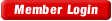Note: If you are a legacy user of chemistry24 members, please request a new login access to the premium server with your full name and old login email via vip@rapidlearningcenter.com

 Rapid Courses Catalog : Physics in 24 Hours Chemistry in 24 Hours Biology in 24 Hours Mathematics in 24 Hours

Weekly Physics Tips:
Want to become a top gun in your class? How about study less yet score high? Sign up this Physics Survival Weekly to learn how. Designed specifically for students who are taking physics, this free newsletter will show you how to survive and excel in class! Weekly topics include:
• How to Study Physics Effectively
• How to Read Physics Textbooks Easily
• How to Solve Physics Problems Systematically
• How to Score High on Physics Exams Strategically
• How to Master Physics Rapidly
Each week, you will receive study tips on the topics above and visual tutorial or study template to enhance your physics learning. Enter your name and email below to subscribe free:

 Physics Study Lounge These study sheets are for quick review on the subjects. Refer to our rapid courses for comprehensive review.     - Basic Skills in Physics     - Basic Math for Physics     - How to Solve Physics Problems     - Newton's Laws Study Guide     - ElectroMagnetism Quick Review     - Atomic Physics At-A-Glance     - Core Concepts in Nuclear Physics     - Special Relativity Overview

 Other Related Sites
 Note: For course links to launch, disable popup blockers or hold the ctrl key while clicking the link.

Basic Math for Physics

Algebra

 If a # is … to a variable, then … the # to solve for the variable Example Added Subtract 5 = x + 2 -2       -2  5-2 = x Subtracted Add 3 = x – 6 +6       +6  3-6 = x Multiplied Divide 2 = 4x  2/4  4x/4 2/4 = x Divided Multiply 2 · 6 = x · 2             2 2 · 6 = x

Calculations with Significant Figures

• Perform the calculation
• Determine the least # of decimal places in problem
• Round answer to that # of decimal places
•         Example:           10.027 g   ® 3 decimal places
•                                  - 1.5     g   ® 1 decimal place
•                                   8.527  g  ® 8.5 g (1 decimal place)
•

Multiplying & Dividing:

• Perform the calculation
• Determine the least # of sig figures in problem
• Round answer to that # of sig figures
•       Example:         10.027 g   ® 5 sig figs
•                                1.50 mL  ® 3 sig figs
• answer = 6.6847 g/mL ® 6.68 g/mL (3 sig digs)

Scientific Notation

• Scientific Notation—a short hand method of writing numbers using powers of 10.
•
• Writing scientific notation:
• The decimal point is always moved to after the 1st non-zero number.
• Count the number of times the decimal point is moved and use this as the power of 10.
• “Big” numbers (>1) have positive exponents.  “Small” numbers (<1) have negative exponents.
•       Examples:      1027500.456 ® 1.027500456 ´ 106
•                            0.0007543 ® 7.543 ´ 104
•
• Power of 10 = number of times to move decimal point
• Positive powers = make the number “Big” (>1).  Negative exponents = make the number “Small” (<1)
• Examples:     3.25 ´ 10-6 ® 0.00000325
• 7.2004 ´ 104 ® 7200.4

Math with Exponents

• Calculations with exponents:

Anything to the power of “1” = itself

•                   251 = 25

Anything to the power of “0” = 1

•                   250 = 1

Multiplying (with the same base) à add the powers

•                   32 * 38 = 311

Dividing (with the same base) à subtract the powers

•                   32 ¸ 38 = 3-6

When taking a power of a power à multiply the powers

•                  (32)3 = 36

A negative power puts the number on the opposite side of the fraction & the power becomes positive.

•                   3-2 = 1/32
•
• Calculations with scientific notation

Adding (with same power of 10):  Add numbers and keep power of 10

•                    2 ´ 103 + 3 ´ 103 = 5 ´ 103

Subtracting (with same power of 10): Subtract numbers and keep power of 10

•                    3 ´ 103 – 3 ´ 103 = -1 ´ 103

Multiplying:  Multiply numbers & add powers of ten

•                    2 ´ 106 · 3 ´ 103 = 6 ´ 1018

Dividing:  Divide numbers & subtract powers of 10

•                    2 ´ 106 ¸ 3 ´ 103 = 0.67 ´ 103

Taking it to a power:  Take the number to the power and multiply the power of 10 by the power

•                   (2 ´ 103)3 = 8 ´ 109

Roots:  Take the number to the root and divide the power of 10 by the root

•                   Ö(3 ´ 102) = Ö3 ´ 101

Trig functions

•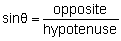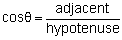•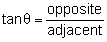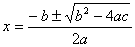•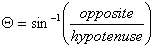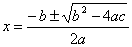whereExample:      IfThen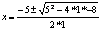Calculator Survival

• Always use the ¸ key to designate a number is on the bottom of an expression.
• Always use the EE (or EXP) key to enter scientific notation.
• Always use parenthesis around addition or subtraction when combining it with other operations
• To make something negative (when taking the number to a power), keep the negative outside of the parenthesis.